# Solution assignment 05 Implicit differentiation

### Assignment 5

Calculate the tangent line at the point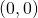of the following graph: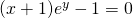### Solution

First we have to verify that the pointlies on the graph, and yes, we have: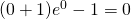Next we have to determine the derivative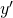and use both the product and quotient rule: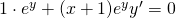and thus: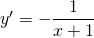and thus in the point: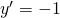The equation of the tangent line becomes: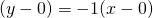or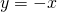.

Also in this case implicit differentiation is not necessary. We can write the function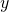in explicit form quite easily: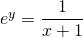and thus, after applying the logarithm in the left- and right-hand side we get: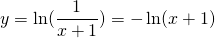Differentiation of this function gives the result found above.